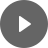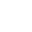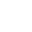8.1 Parabola - StudyNinja
Select Page

HSC Standard Maths Resources

Browse: 1. Home  »  8. Non-linear Relationship »  8.1 Parabola

8.1 Parabola

Parabola is a curve that is formed from a quadratic equation. Equations where the highest power of x is 2 are called quadratic equations.Example 1

Draw the graph of quadratic equation y = x2 – 3x + 5. What is the minimum point? What is the axis of symmetry? What are the x and y-intercepts?

Example 2

Which one of the following equations best describe the graph below?Get access to 20 Mock Exams with over 700 exam-style questions for HSC Standard Maths.

You might find the following videos helpful related to this section:8C Quadratic Functions (1 of 4)

by Mr Bodgers (click to view channel)

Explains what a quadratic function and a parabola looks like.8C Quadratic Functions (2 of 4)

by Mr Bodgers (click to view channel)

Completes an example where a quadratic functions is plotted on a graph using a table of values.8C Quadratic Functions (3 of 4)

by Mr Bodgers (click to view channel)

Uses Desmos to explain how to perform transformations on the basic parabola.8C Quadratic Functions (4 of 4)

by Mr Bodgers (click to view channel)

This is an interactive graphing activity. You are asked to create 5 different parabolas that pass through a series of points.8D Quadratic Models (1 of 2)

by Mr Bodgers (click to view channel)

This example uses a quadratic function to model a practical problem.8D Quadratic Models (2 of 2)

by Mr Bodgers (click to view channel)

Completes an example on a cars stopping distance using a quadratic function.The following are the types of questions you can expect in exam:

Study notes of this section and other resources can be accessed here:

Browse: 1. Home  »  8. Non-linear Relationship »  8.1 Parabola